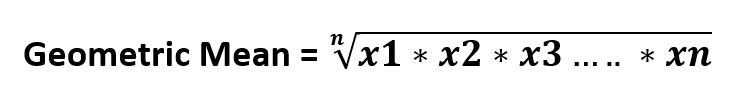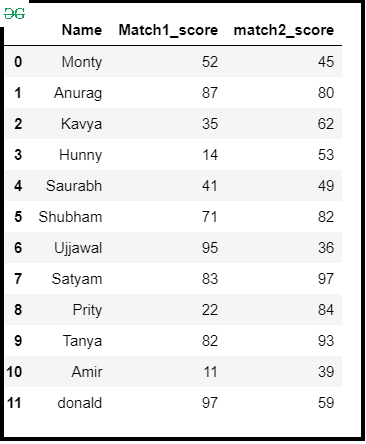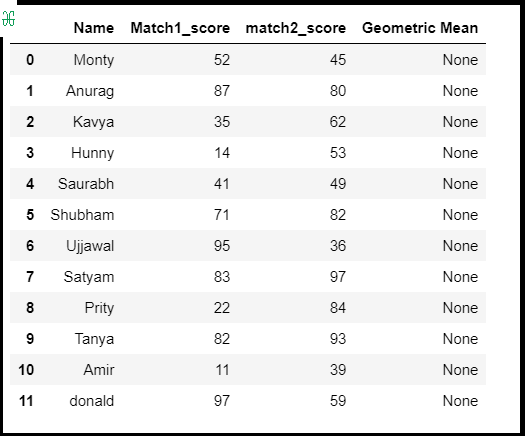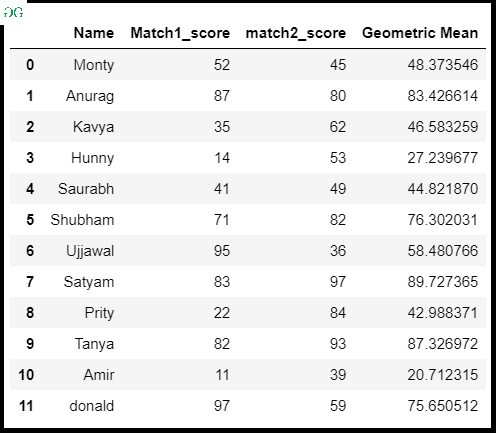# Find the geometric mean of a given Pandas DataFrame

## Find the geometric mean of a given Pandas DataFrame

In this article, we will discuss how to find the geometric mean of a given DataFrame. Generally geometric mean of nth numbers is the nth root of their product.It can found using the scipy.stats.gmean() method. This function calculates the geometric mean of the array elements along the specified axis of the array (list in python).

Syntax:

`scipy.stats.gmean(array, axis=0, dtype=None)`

Approach :

• Import module
• Create Pandas DataFrame
• Create a new column for the geometric mean
• Find the geometric mean with scipy.stats.gmean()
• Store into a new column
• Display DataFrame

Step-by-Step Implementation :

Step 1: Importing module and Making Dataframe.

 `# importing module` `import` `pandas as pd` `import` `numpy as np` `from` `scipy ``import` `stats` ` ` `# Create a DataFrame` `df ``=` `pd.DataFrame({` `    ``'Name'``: [``'Monty'``, ``'Anurag'``, ``'Kavya'``, ``'Hunny'``, ``'Saurabh'``,` `             ``'Shubham'``, ``'Ujjawal'``, ``'Satyam'``, ``'Prity'``, ``'Tanya'``, ` `             ``'Amir'``, ``'donald'``],` `    ``'Match1_score'``: [``52``, ``87``, ``35``, ``14``, ``41``, ``71``, ``95``, ``83``, ``22``, ``82``, ``11``, ``97``],` `    ``'match2_score'``: [``45``, ``80``, ``62``, ``53``, ``49``, ``82``, ``36``, ``97``, ``84``, ``93``, ``39``, ``59``]})` ` ` `# Display DataFrame` `df`

Output :Step 2:  Create an empty DataFrame column.

 `# Creating empty column in DataFrame` `df[``'Geometric Mean'``] ``=` `None` `df`

Output :Step 3: Find Geometric mean with scipy.stats.gmean()  and store it into a new column.

 `# Computing geometric mean` `# Storing into a DataFrame column` `df[``'Geometric Mean'``] ``=` `stats.gmean(df.iloc[:, ``1``:``3``], axis``=``1``)` `df`

Output :Last Updated on October 23, 2021 by admin

## Pandas Series.plot() methodPandas Series.plot() method

Python | Pandas Series.plot() method With the help of Series.plot() method, we can get the plot of

## Sort Correlation Matrix in PythonSort Correlation Matrix in Python

Sort Correlation Matrix in Python A correlation matrix investigates the dependence between multiple variables at

## Pandas dataframe.set_value()Pandas dataframe.set_value()

Pandas dataframe.set_value()   Pandas dataframe.set_value() function put a single value at passed column and index.

## Extract week number from date in Pandas-PythonExtract week number from date in Pandas-Python

Extract week number from date in Pandas-Python Many times, when working with some data containing

## Ways to apply an if condition in Pandas DataFrameWays to apply an if condition in Pandas DataFrame

Ways to apply an if condition in Pandas DataFrame Generally on a Pandas DataFrame the

## Rendering Data-Frame to html template in table view using Django FrameworkRendering Data-Frame to html template in table view using Django Framework

Rendering Data-Frame to html template in table view using Django Framework n Django, It is

## How to List values for each Pandas group?How to List values for each Pandas group?

How to List values for each Pandas group? In this article, we’ll see how we

## Create pandas dataframe from lists using zipCreate pandas dataframe from lists using zip

Create pandas dataframe from lists using zip One of the way to create Pandas DataFrame